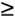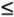I

 [Page 790 ( continued )] identity matrix a matrix containing ones along the diagonal and zeros elsewhere implementation the use of model results implicit enumeration a method for solving integer programming problems in which obviously infeasible solutions are eliminated and the remaining solutions are systematically evaluated to see which one is best independent demand final product demanded by an external customer independent events events for which the probability of occurrence of one event does not affect the probability of occurrence of the other events [Page 791] inequality a mathematical relationship containing aorsign infeasible problem a linear programming problem with no feasible solution area and thus no solution instantaneous receipt the assumption that once inventory level reaches zero, an order is received after the passage of an infinitely small amount of time integer programming a form of linear programming that generates only integer solution values for the model variables inventory a stock of items kept on hand by an organization to use to meet customer demand inventory analysis the analysis of the problems of inventory planning and control, with the objective of minimizing inventory- related costsIntroduction to Management Science (10th Edition)
ISBN: 0136064361
EAN: 2147483647
Year: 2006
Pages: 358

Similar book on Amazon